### Picard groups of curves over non-algebraically closed fields

Posted on June 5, 2019

If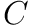is a curve defined over a field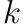we generally define the divisor group of, denoted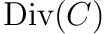, as the free abelian group generated by points in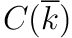. We then define the principal divisors as the subgroup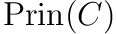ofconsisting of all divisors of the form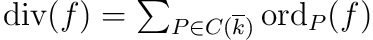with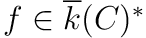. We then define the Picard group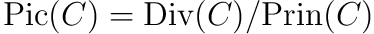.

Given a divisor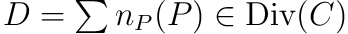and an automorphism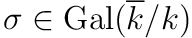we define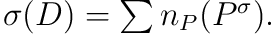We say that a divisor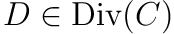is defined over, denoted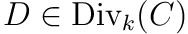, if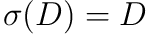for all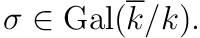We can then similarly define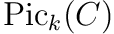to be the set of classes of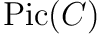that are invariant under every element of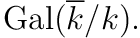From the above definitions we have the following exact sequence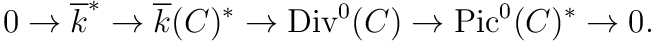We would hope to have another exact sequence for the corresponding objects defined over. In fact we will show that we always have an exact squence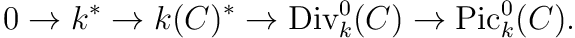It is immediate that the above sequence is exact at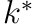and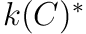, but it is surprisingly non-elementary to observe that the sequence is exact at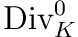. In order to show that the sequence is exact atwe need to show that if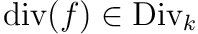for some, then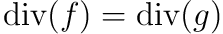for some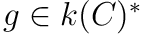. That is, we need to show that we have the following short exact sequence: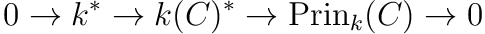where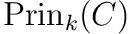is the subset of Galois invariant divisors in. This is precisely the situation that is studied by Galois cohomology. We have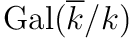-modules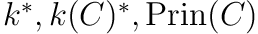, and a short exact sequence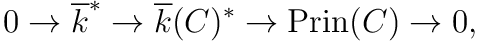and we wish to know if exactness holds once consider only the submodules invariant under. Galois cohomology, or more generally group cohomology, provides a long exact sequence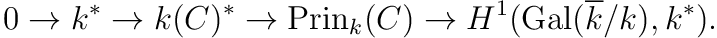Finally, the cohomological version of Hilbert’s Theorem 90 tells us that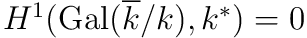.

The inspiration for this problem comes from Silverman (1, Exercise 2.13).

1. Joseph Silverman, The arithmetic of elliptic curves, 2nd ed., Springer Graduate Texts in Mathematics, 1992